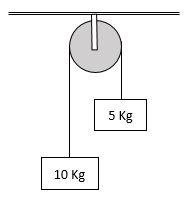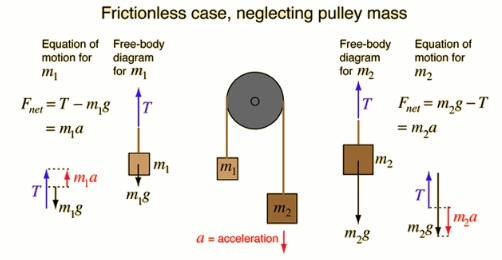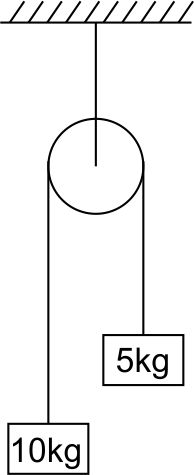#### Two blocks of masses 5kg and 10kg are connected to a pulley as shown in fig. What will be the acceleration if the pulley is set free?Option 1) g Option 2) g/2 Option 3) g/3 Option 4) g/4As we learnt in

Motion of connected blocks over pulley -Equation of motion forEquation of Motion for- whereinOption 1)

g

Incorrect

Option 2)

g/2

Incorrect

Option 3)

g/3

Correct

Option 4)

g/4

Incorrect

#### prateek

(M1-M2)G/M1+M2.     =10-5×g/10+5=5g/15=g/3

Option 3